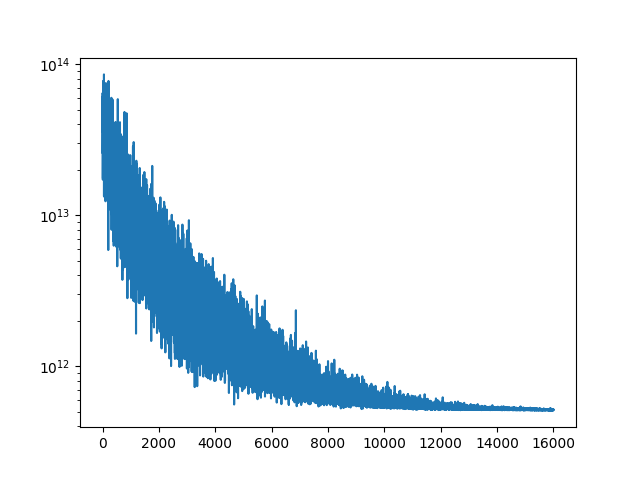# Infered variance low

I’m playing around with a toy problem, representative of a real problem I’m trying to solve. Say we have a canon positioned to fire a projectile at some angle and velocity and we can measure the resulting trajectory. The canon has a wobble or something so the initial angle and velocity are uncertain. I’m trying to infer the uncertainty in both by observing a number of trajectories.

The working code below simulates some number of trajectories using known normal distributions for the angle and velocity. For compatibility with my actual problem the trajectories are stored as a n_time x n_batch x 2 tensor. The code then runs a MAP estimate for the mean values of the actual distributions and then SVI to try to find both the mean and variance.

The MAP estimate is fine, with these settings it recovers the mean of the input distributions. SVI again recovers the mean but, as the final plots that the code generates shows, the estimate of the variance of both the angle and velocity distributions is too low. This is very consistent no matter how I set the prior or the initial (AutoNormal) scale parameters. For example, even if I set the initial guide variances to be high the inference process will still tend to send them towards zero.

Is there something fundamentally wrong with this approach or does anyone have any suggestions on how to improve the variance predictions?

``````import numpy as np
import matplotlib.pyplot as plt

import torch
import pyro
import pyro.distributions as dist
from pyro.contrib.autoguide import AutoDiagonalNormal, AutoDelta, AutoNormal, init_to_mean
from pyro.infer import SVI, Trace_ELBO, Predictive
import pyro.optim as optim

from tqdm import tqdm

g = torch.tensor(0.1)

v_loc_act = 2.0
v_scale_act = 0.1
a_loc_act = np.pi/6.0
a_scale_act = 0.02

v_loc_prior = 1.5
v_scale_prior = 0.2
a_loc_prior = np.pi/4
a_scale_prior = 0.04

eps = 1.0e-4

pyro.enable_validation()

def model_act(times):
"""
times: ntime x nbatch
trajectories: ntime x nbatch x 2
"""
v = pyro.sample("v", dist.Normal(v_loc_act, v_scale_act))
a = pyro.sample("a", dist.Normal(a_loc_act, a_scale_act))

simulated = torch.stack((
v * torch.cos(a) * times,
v * torch.sin(a) * times - 0.5 * g * times**2.0)).T

return simulated

def model(times, actual = None):
"""
times: ntime x nbatch
trajectories: ntime x nbatch x 2
"""
v = pyro.sample("v", dist.Normal(v_loc_prior, v_scale_prior))
a = pyro.sample("a", dist.Normal(a_loc_prior, a_scale_prior))

simulated = torch.stack((
v * torch.cos(a) * times,
v * torch.sin(a) * times - 0.5 * g * times**2.0)).permute((1,2,0))

with pyro.plate("trials", times.shape):
with pyro.plate("time", times.shape):
pyro.sample("obs", dist.Normal(simulated, eps).to_event(1), obs = actual)

return simulated

if __name__ == "__main__":
nsamples = 50

tmax = 20.0
tnum = 100

time = torch.linspace(0, tmax, tnum)
times = torch.empty(tnum, nsamples)
data = torch.empty(tnum, nsamples, 2)

for i in range(nsamples):
times[:,i] = time
data[:,i] = model_act(time)

plt.plot(data[:,:,0], data[:,:,1])
plt.show()

# MAP
print("MAP")
pyro.clear_param_store()

lr = 1.0e-3
niter = 1250
num_samples = 1

guide = AutoDelta(model, init_loc_fn = init_to_mean())

svi = SVI(model, guide, optimizer,
loss = Trace_ELBO(num_particles=num_samples))

t = tqdm(range(niter))
loss_hist = []
for i in t:
loss = svi.step(times, data)
loss_hist.append(loss)
t.set_description("Loss: %3.2e" % loss)

print("MAP velocity: %4.3f, actual %4.3f" % (pyro.param("AutoDelta.v").data,
v_loc_act))
print("MAP angle: %4.3f, actual %4.3f" % (pyro.param("AutoDelta.a").data,
a_loc_act))

plt.semilogy(loss_hist)
plt.show()

print("")

# Inference with AutoNormal
pyro.clear_param_store()

print("AutoNormal")

lr = 1.0e-3
niter = 8000
num_samples = 5

guide = AutoNormal(model, init_loc_fn = init_to_mean(),
init_scale = 0.1)
# Initialize the guide
guide(times, data)

svi = SVI(model, guide, optimizer,
loss = Trace_ELBO(num_particles=num_samples))

print("Start:")
print("Velocity mean: %4.3f, actual %4.3f" % (pyro.param("AutoNormal.locs.v").data,
v_loc_act))
print("Velocity scale: %4.3f, actual %4.3f" % (pyro.param("AutoNormal.scales.v").data,
v_scale_act))
print("Angle mean: %4.3f, actual %4.3f" % (pyro.param("AutoNormal.locs.a").data,
a_loc_act))
print("Angle scale: %4.3f, actual %4.3f" % (pyro.param("AutoNormal.scales.a").data,
a_scale_act))

t = tqdm(range(niter))
loss_hist = []
for i in t:
loss = svi.step(times, data)
loss_hist.append(loss)
t.set_description("Loss: %3.2e" % loss)

print("Inference:")
print("Velocity mean: %4.3f, actual %4.3f" % (pyro.param("AutoNormal.locs.v").data,
v_loc_act))
print("Velocity scale: %4.3f, actual %4.3f" % (pyro.param("AutoNormal.scales.v").data,
v_scale_act))
print("Angle mean: %4.3f, actual %4.3f" % (pyro.param("AutoNormal.locs.a").data,
a_loc_act))
print("Angle scale: %4.3f, actual %4.3f" % (pyro.param("AutoNormal.scales.a").data,
a_scale_act))

plt.semilogy(loss_hist)
plt.show()

nsample = 200
predict = Predictive(model, guide = guide, num_samples = nsample,
return_sites=("obs",))
samples = predict(times[:,0:1])["obs"][:,:,0]
min_x, _ = torch.min(samples[:,:,0], 0)
max_x, _ = torch.max(samples[:,:,0], 0)
min_y, _ = torch.min(samples[:,:,1], 0)
max_y, _ = torch.max(samples[:,:,1], 0)

plt.plot(times, data[:,:,1], 'k-', lw = 0.1)
plt.fill_between(time, min_y, max_y, alpha = 0.75)
plt.show()

plt.plot(times, data[:,:,0], 'k-', lw = 0.1)
plt.fill_between(time, min_x, max_x, alpha = 0.75)
plt.show()``````

variational inference has a general tendency to underestimate variances/uncertainty. you might get better results if you use a `AutoMultivariateNormal` guide. alternatively you could use HMC, which should give you nearly perfect draws from the posterior, at least in this particular problem, which is quite simple

Thanks. AutoMultivariateNormal doesn’t seem to be much more effective, see the loss history below. Apparently SVI is going to tend to damp out any initial variance I provide the guide, at least for this problem. In fact it seems to approach zero variance over time, which is why I was wondering if I was structuring something wrong.HMC is pretty effective for these problems.

please note that your model has a single pair of global latent variables (v and a); in particular they are outside the plates and shared for all observations.

on the other hand your simulated data is drawn with many different values of v and a. if you want to infer distinct v’s and a’s for each trajectory then you need to move your latents into the plate. you could then make your model hierarchical by having e.g. the means of the a_i and v_i be shared global latent variables. i think this is what you want given your description.

Yep, that’s the problem. Thanks for the help.